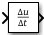# Derivative

Output time derivative of input

• Library:
• Simulink / Continuous

•## Description

The Derivative block approximates the derivative of the input signal u with respect to the simulation time t. You obtain the approximation of

`$\frac{du}{dt},$`

by computing a numerical difference $\Delta u/\Delta t,$where $\Delta u$ is the change in input value and $\Delta t$ is the change in time since the previous simulation (major) time step.

This block accepts one input and generates one output. The initial output for the block is zero.

The precise relationship between the input and output of this block is:

`$y\left(t\right)=\frac{\Delta u}{\Delta t}=\frac{u\left(t\right)-u\left({T}_{previous}\right)}{t-{T}_{previous}}|t>{T}_{previous},$`

where t is the current simulation time and ${T}_{previous}$ is the time of the last output time of the simulation. The latter is the same as the time of the last major time step.

The Derivative block output might be sensitive to the dynamics of the entire model. The accuracy of the output signal depends on the size of the time steps taken in the simulation. Smaller steps allow for a smoother and more accurate output curve from this block. However, unlike with blocks that have continuous states, the solver does not take smaller steps when the input to this block changes rapidly. Depending on the dynamics of the driving signal and model, the output signal of this block might contain unexpected fluctuations. These fluctuations are primarily due to the driving signal output and solver step size.

Because of these sensitivities, structure your models to use integrators (such as Integrator blocks) instead of Derivative blocks. Integrator blocks have states that allow solvers to adjust the step size and improve simulation accuracy. See Circuit Model for an example of choosing the best-form mathematical model to avoid using Derivative blocks in your models.

If you must use the Derivative block with a variable step solver, set the solver maximum step size to a value such that the Derivative block can generate answers with adequate accuracy. To determine this value, you might need to repeatedly run the simulation using different solver settings.

If the input to this block is a discrete signal, the continuous derivative of the input exhibits an impulse when the value of the input changes. Otherwise, it is 0. Alternatively, you can define the discrete derivative of a discrete signal using the difference of the last two values of the signal:

`$y\left(k\right)=\frac{1}{\Delta t}\left(u\left(k\right)-u\left(k-1\right)\right)$`

.

Taking the z-transform of this equation results in:

`$\frac{Y\left(z\right)}{u\left(z\right)}=\frac{1-{z}^{-1}}{\Delta t}=\frac{z-1}{\Delta t\cdot z}.$`

The Discrete Derivative block models this behavior. Use this block instead of the Derivative block to approximate the discrete-time derivative of a discrete signal.

## Ports

### Input

expand all

Signal to be differentiated, specified as a real scalar or vector.

Data Types: `double`

### Output

expand all

Time derivative of input signal, specified as a real scalar or vector. The input signal is differentiated with respect to time as:

`$y\left(t\right)=\frac{\Delta u}{\Delta t}=\frac{u\left(t\right)-u\left({T}_{previous}\right)}{t-{T}_{previous}}|t>{T}_{previous},$`

where t is the current simulation time and ${T}_{previous}$ is the time of the last output time of the simulation. The latter is the same as the time of the last major time step.

Data Types: `double`

## Parameters

expand all

The exact linearization of the Derivative block is difficult because the dynamic equation for the block is $y=\stackrel{˙}{u}$, which you cannot represent as a state-space system. However, you can approximate the linearization by adding a pole to the Derivative block to create a transfer function $s/\left(c\ast s+1\right).$ The addition of a pole filters the signal before differentiating it, which removes the effect of noise.

The default value `inf` corresponds to a linearization of `0`.

#### Tips

• As a best practice, change the value of c to $\frac{1}{{f}_{b}}$, where ${f}_{b}$ is the break frequency of the filter.

• The parameter must be a finite positive value.

#### Programmatic Use

 Block Parameter: `CoefficientInTFapproximation` Type: character vector, string Values: `'inf'` Default: `'inf'`

## Block Characteristics

 Data Types `double` Direct Feedthrough `no` Multidimensional Signals `no` Variable-Size Signals `no` Zero-Crossing Detection `no`

## Version History

Introduced before R2006a